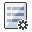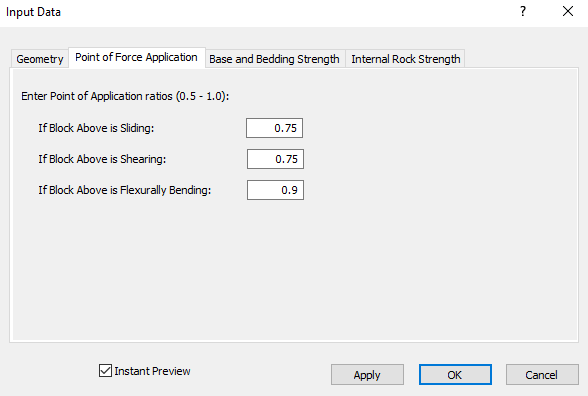The Rocscience International Conference 2021 Proceedings are now available. Read Now

# Point of Force Application

The Point of Force Application ratios used in a RocTopple analysis are entered in the Point of Force Application tab in the Project Settings dialog. To open the tab:

1. Select Input Dataon the toolbar or the Analysis menu or press F3.
2. Select the Point of Force Application tab.Point of Force Application is the location where the normal force (Pn) due to the failure of the block above (n+1) is applied on block n. Since the block above has the potential for toppling, sliding, toppling-sliding, or remaining stable, you can enter a different ratio for each. The ratio is multiplied to the height of the joint with the block above (hn). Valid inputs range from 0.5 to 1. The default values in the tab are:

• If Block Above is Sliding = 0.75
• If Block Above is Shearing = 0.75
• If Block Above is Flexurally Bending = 0.9 (only available for the Block Flexure Toppling method)

NOTE: If the block above is stable, Pn is zero. In this case, the Point of Force Application is inconsequential.